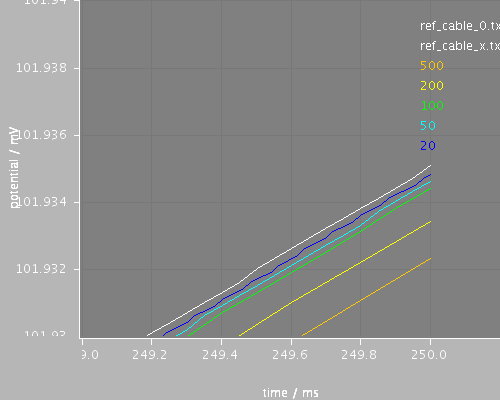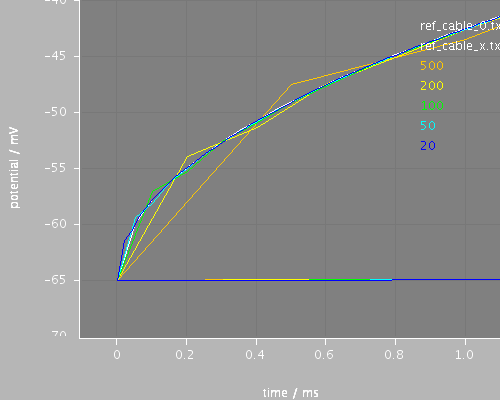## ralpack-dev (runwcn.xml)

Cable, 100um long, run for 250ms with different timesteps between 20 and 500 microseconds

Uniform unbranched cable. The task specification and reference results come from Upi Bhalla's 1992 Rallpack distribution, part of which is included below. The rest in the file README.upi.

The model produces three graphs showing the reference data (correct solution) in white, and calculated curves in other colors according to the timestep used. The only difference between the graphs are the axis ranges: they all show the same data.

If the system performs correctly, the results should be almost superimposed with less than 0.01 mV difference from the reference, for any of the timesteps.

From Upi Bhalla's 1992 Rallpack package
```This simulation models a simple uniform unbranched cable with
1000 identical compartments, with a length constant of 1, a diameter
of 1 micron, and a total length of 1 mm. The membrane properties are :

RA = 1.0 ohms meter             = 100 ohms cm
RM = 4.0 ohms meter^2           = 40000 ohms cm^2
CM = 0.01 Farads/meter^2        = 1.0 uf/cm^2
EM = -0.065 Volts               = -65 mV
ELEAK = EREST = EM

A current of 0.1 nA is injected in the first compartment. Membrane voltages
are recorded for the first and last compartments.
This model is run for a simulated time of 0.25 seconds.

```

Total CPU time 3.095 seconds; at 17:20:58 Mon 3 Sep 2007

 Compartments Stochasticchannels / cpmts Continuouschannels / cpmts time/ms timeStep CPU Time / s 1002 0 / 0 0 / 0 250.0 20 1.744 1002 0 / 0 0 / 0 250.0 50 0.709 1002 0 / 0 0 / 0 250.0 100 0.362 1002 0 / 0 0 / 0 250.0 200 0.192 1002 0 / 0 0 / 0 250.0 500 0.088### Predefined views

#### whole#### end#### midrange#### startts-500-f.txt, line 0, deviation: mean = -0.0377, rms= 0.101
ts-500-f.txt, line 1, deviation: mean = -0.0268, rms= 0.0424
ts-200-f.txt, line 0, deviation: mean = -0.0213, rms= 0.0450
ts-200-f.txt, line 1, deviation: mean = -0.0161, rms= 0.0233
ts-100-f.txt, line 0, deviation: mean = -0.00695, rms= 0.0205
ts-100-f.txt, line 1, deviation: mean = -0.00422, rms= 0.00725
ts-50-f.txt, line 0, deviation: mean = -0.00451, rms= 0.0110
ts-50-f.txt, line 1, deviation: mean = -0.00307, rms= 0.00463
ts-20-f.txt, line 0, deviation: mean = -0.00182, rms= 0.0140
ts-20-f.txt, line 1, deviation: mean = -0.00141, rms= 0.00201

## All files

 Model Preprocessed Outupt data Reference data etc runwcn.xml cell.xml membrane.xml environment.xml recording.xml leak.xml summary.xml view.xml ts-20-fdata.txt ts-50-fdata.txt ts-100-fdata.txt ts-200-fdata.txt ts-500-fdata.txt ts-20-f.dat ts-20-f.txt ts-50-f.dat ts-50-f.txt ts-100-f.dat ts-100-f.txt ts-200-f.dat ts-200-f.txt ts-500-f.dat ts-500-f.txt ref_cable_0.txt ref_cable_x.txt start.txt README README.upi ralpack-dev.jar

## Model

Archive file of the complete model: ralpack-dev.jar

### runwcn.xml

```<PSICSRun timeStep="0.1ms" runTime="250ms" startPotential="-65mV"

tdWeighting="0.65"
morphology="cell"
environment="environment"
properties="membrane"
access="recording">

<StructureDiscretization baseElementSize="1um"/>

<RunSet vary="timeStep" values="[20, 50, 100, 200, 500]us"
filepattern="ts-\$.txt"/>
<info>Cable, 100um long, run for 250ms with different timesteps between 20 and 500 microseconds</info>

<ViewConfig>
<LineGraph width="500" height="400">
<XAxis min="0" max="250" label="time / ms"/>
<YAxis min="-80" max="60" label="potential / mV"/>
<Line file="ref_cable_0.txt" color="white" width="2" rescale="[1000, 1000.]"/>
<Line file="ref_cable_x.txt" color="white" width="2" rescale="[1000, 1000.]"/>

<LineSet file="ts-500-f.txt" color="orange" label="500">
<DataComparison file="ref_cable_0.txt" line="0"rescale="[1000, 1000.]"/>
<DataComparison file="ref_cable_x.txt" line="1"rescale="[1000, 1000.]"/>
</LineSet>

<LineSet file="ts-200-f.txt" color="yellow" label="200">
<DataComparison file="ref_cable_0.txt" line="0" rescale="[1000, 1000.]"/>
<DataComparison file="ref_cable_x.txt" line="1" rescale="[1000, 1000.]"/>
</LineSet>

<LineSet file="ts-100-f.txt" color="green" label="100">
<DataComparison file="ref_cable_0.txt" line="0" rescale="[1000, 1000.]"/>
<DataComparison file="ref_cable_x.txt" line="1" rescale="[1000, 1000.]"/>
</LineSet>

<LineSet file="ts-50-f.txt" color="cyan" label="50">
<DataComparison file="ref_cable_0.txt" line="0" rescale="[1000, 1000.]"/>
<DataComparison file="ref_cable_x.txt" line="1" rescale="[1000, 1000.]"/>
</LineSet>

<LineSet file="ts-20-f.txt" color="blue" label="20">
<DataComparison file="ref_cable_0.txt" line="0" rescale="[1000, 1000.]"/>
<DataComparison file="ref_cable_x.txt" line="1" rescale="[1000, 1000.]"/>
</LineSet>

<View id="whole" xmin="-10." xmax="260." ymin="-100." ymax="100."/>
<View id="end" xmin="249" xmax="250.2" ymin="101.93" ymax="101.94"/>
<View id="midrange" xmin="80" xmax="90" ymin="25" ymax="30"/>

<View id="start" xmin="-0.1" xmax = "1.1" ymin = "-70" ymax="-40"/>

</LineGraph>
</ViewConfig>

</PSICSRun>
```

### cell.xml

```<CellMorphology id="cell">
<Point id="p0" x="0" y="0" z="0" r="0.5"/>
<Point parent="p0" id="p1" x="1000" y="0" z = "0" r="0.5"/>
</CellMorphology>
```

### membrane.xml

```<CellProperties id="membrane"
cytoplasmResistivity="100ohm_cm"
membraneCapacitance="1uF_per_cm2">

<ChannelPopulation channel="leak" density="25per_um2"/>

</CellProperties>
```

### environment.xml

```<CellEnvironment id="environment">
<Ion id="K" name="Potassium" reversalPotential="-65mV"/>
</CellEnvironment>
```

### recording.xml

```<Access id="recording">

<CurrentClamp at="p0" lineColor="red" hold="0.1nA">

</CurrentClamp>

<VoltageRecorder at="p1" lineColor="blue"/>

</Access>
```

### leak.xml

```<KSChannel id="leak" gSingle="0.01pS" permeantIon="K">
<OpenState id="o1"/>
</KSChannel>
```